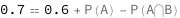# Probability of the Union of Two Events

The probability of the union of two events depends on the probability of either event and the probability of only one of the events occuring.

The probability of A\[Union]B equals the sum of the probabilities of A and B minus the probability of A\[Intersection]B.

## Formula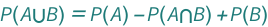symbol description physical quantity
P(A⋃B)
probability of AB
"Unitless"
P(A)
probability of A
"Unitless"
P(A⋂B)
probability of AB
"Unitless"
P(B)
probability of B
"Unitless"

## Examples

Get the resource:

 In:=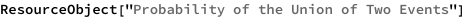Out=Get the formula:

 In:=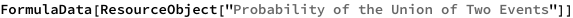Out=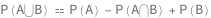Use some values:

 In:=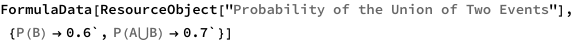Out=